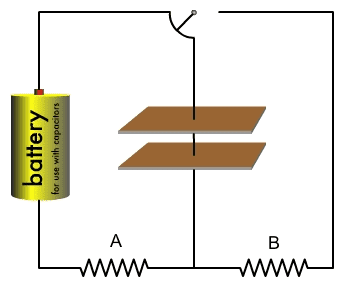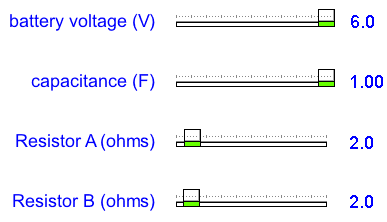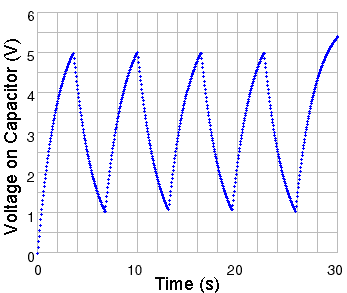# Voltage across resistors in RC circuit

## Homework Statement

Calculate the voltage, current, and power across the two resistors for every second of run 1 in the RC circuit.

The RC Circuit:The settings of the circuit:The Graph. The capacitor is charged until it reaches 5.0 V and then discharged until it reaches 1.0 V. This process occurs four times total.## Homework Equations

VC = ξ * (1 - e-t/RC)

## The Attempt at a Solution

This is a lab more so than a question and therefore doesn't necessarily have an exact answer. I am having trouble with the process of getting an answer.

I have eyeballed the voltages from the graph for every second but am unsure how to calculate the voltage across each resistor. Is the voltage in each resistor the same as the voltage that is currently in the capacitor at time that time s?

I tried calculating the time constant by plugging the capacitor voltage at 5 seconds with a emf of 6.0 V and got 2.79 seconds, but 63% of 6.0 V is 3.78 V and eyeballing the graph I get approx. 2 seconds to reach 63% max voltage.

I feel like I am overthinking this and am unconfident in my knowledge of this section in my textbook, but rereading isn't helping me either.

Simon Bridge
Homework Helper
...am unsure how to calculate the voltage across each resistor. Is the voltage in each resistor the same as the voltage that is currently in the capacitor at time that time s?
No.
The each resistor is connected to the capacitor alternately (A-B-A-...) - so one resistor will have zero volts while the other has some non-zero voltage.
The voltage across the resistor and that of the capacitor, when they are connected, must add up to something.

I tried calculating the time constant by plugging the capacitor voltage at 5 seconds with a emf of 6.0 V and got 2.79 seconds, but 63% of 6.0 V is 3.78 V and eyeballing the graph I get approx. 2 seconds to reach 63% max voltage.
... the time constant is RC=2secs. Don't know what you were doing.

I feel like I am overthinking this and am unconfident in my knowledge of this section in my textbook, but rereading isn't helping me either.
You need to take another look at the circuit - maybe redrawing it to separate the charging and discharging stages - leaving out the components that do not contribute. It should become clear.

No.
The each resistor is connected to the capacitor alternately (A-B-A-...) - so one resistor will have zero volts while the other has some non-zero voltage.
The voltage across the resistor and that of the capacitor, when they are connected, must add up to something.

... the time constant is RC=2secs. Don't know what you were doing.

You need to take another look at the circuit - maybe redrawing it to separate the charging and discharging stages - leaving out the components that do not contribute. It should become clear.

Thanks, I was looking at the circuit wrong (I thought the charge was coming out the top and hitting the resistor after the capacitor).

Is there a way to calculate the voltage of the capacitor as it is discharging from 5V?

Simon Bridge